# 单量子比特门

$$|q\rangle = \cos{\tfrac{\theta}{2}}|0\rangle + e^{i\phi}\sin{\tfrac{\theta}{2}}|1\rangle$$

$θ$和$φ$是实数。在本节中，我们将介绍门，即在这些状态之间更改量子比特的操作。由于门的数量和它们之间的相似性，本章有可能成为列表。为了解决这个问题，我们包括了一些题外话，在整个章节的适当位置介绍重要的想法。

## 目录

[toc]

from qiskit import QuantumCircuit, assemble, Aer
from math import pi, sqrt
from qiskit.visualization import plot_bloch_multivector, plot_histogram
sim = Aer.get_backend('aer_simulator')


## 1.泡利门

### 1.1 X-门

X门由泡利-X矩阵表示：

$$X = \begin{bmatrix} 0 & 1 \ 1 & 0 \end{bmatrix} = |0\rangle\langle1| + |1\rangle\langle0|$$

$$X|0\rangle = \begin{bmatrix} 0 & 1 \ 1 & 0 \end{bmatrix}\begin{bmatrix} 1 \ 0 \end{bmatrix} = \begin{bmatrix} 0 \ 1 \end{bmatrix} = |1\rangle$$

$$M|v\rangle = \begin{bmatrix}a & b \ c & d \\end{bmatrix}\begin{bmatrix}v_0 \ v_1 \\end{bmatrix} = \begin{bmatrix}a\cdot v_0 + b \cdot v_1 \ c \cdot v_0 + d \cdot v_1\end{bmatrix}$$

$$X = |0\rangle\langle1| + |1\rangle\langle0|$$

\begin{aligned} X|1\rangle & = (|0\rangle\langle1| + |1\rangle\langle0|)|1\rangle \ & = |0\rangle\langle1|1\rangle + |1\rangle\langle0|1\rangle \ & = |0\rangle \times 1 + |1\rangle \times 0 \ & = |0\rangle \end{aligned}

事实上，当我们看到一个 ket 和一个bra像这样乘以时：


$$|a\rangle\langle b|$$

$$|a\rangle\langle b| = \begin{bmatrix} a_0 b_0^* & a_0 b_1^* & \dots & a_0 b_n^\ a_1 b_0^ & \ddots & & \vdots \ \vdots & & \ddots & \vdots \ a_n b_0^* & \dots & \dots & a_n b_n^* \ \end{bmatrix}$$

$∗$表示复共轭。我们可以看到，这确实会产生上面所示的x矩阵:
$$|0\rangle\langle1| + |1\rangle\langle0| = \begin{bmatrix}0 & 1 \ 0 & 0 \\end{bmatrix} + \begin{bmatrix}0 & 0 \ 1 & 0 \\end{bmatrix} = \begin{bmatrix}0 & 1 \ 1 & 0 \\end{bmatrix} = X$$

# Let's do an X-gate on a |0> qubit
qc = QuantumCircuit(1)
qc.x(0)
qc.draw()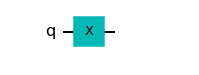# Let's see the result
qc.save_statevector()
qobj = assemble(qc)
state = sim.run(qobj).result().get_statevector()
plot_bloch_multivector(state)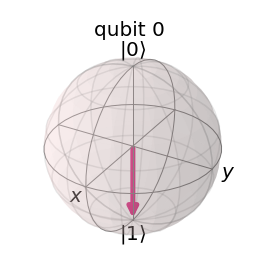### 1.2 Y&Z-门

$$Y = \begin{bmatrix} 0 & -i \ i & 0 \end{bmatrix} \quad\quad\quad\quad Z = \begin{bmatrix} 1 & 0 \ 0 & -1 \end{bmatrix}$$

$$Y = -i|0\rangle\langle1| + i|1\rangle\langle0| \quad\quad Z = |0\rangle\langle0| - |1\rangle\langle1|$$

# Run the code in this cell to see the widget
from qiskit_textbook.widgets import gate_demo
gate_demo(gates='pauli')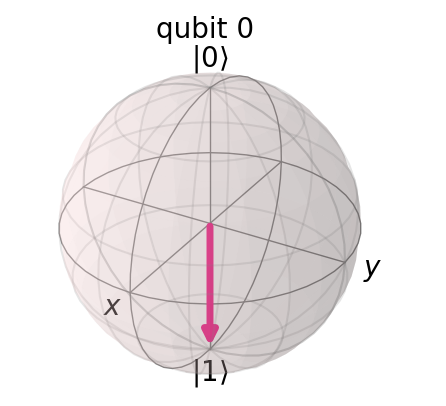qc.y(0) # Do Y-gate on qubit 0
qc.z(0) # Do Z-gate on qubit 0
qc.draw()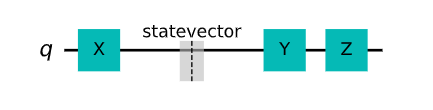## 2.题外话：X、Y&Z基

$$M|v\rangle = |v’\rangle \leftarrow \text{new vector}$$

$$M|v\rangle = \lambda|v\rangle$$

(上面，$M$是一个矩阵，$λ$是一个标量)。对于矩阵$M$，任何具有这个性质的向量称为M的特征向量。例如，Z-矩阵的特征向量是状态$|0⟩$和$|1⟩$:

\begin{aligned} Z|0\rangle & = |0\rangle \ Z|1\rangle & = -|1\rangle \end{aligned}

$$|+\rangle = \tfrac{1}{\sqrt{2}}(|0\rangle + |1\rangle) = \tfrac{1}{\sqrt{2}}\begin{bmatrix} 1 \ 1 \end{bmatrix}$$

$$|-\rangle = \tfrac{1}{\sqrt{2}}(|0\rangle - |1\rangle) = \tfrac{1}{\sqrt{2}}\begin{bmatrix} 1 \ -1 \end{bmatrix}$$

$$|\circlearrowleft\rangle, \quad |\circlearrowright\rangle$$

#### 快速练习

1.验证$|+⟩$和$|-⟩$实际上是X门的本征态。

2.它们有什么特征值?

3.找到Y门的本征态，以及它们在布洛赫球面上的坐标。

## 3.哈达玛门

$$H = \tfrac{1}{\sqrt{2}}\begin{bmatrix} 1 & 1 \ 1 & -1 \end{bmatrix}$$

$$H|0\rangle = |+\rangle$$

$$H|1\rangle = |-\rangle$$

# Run the code in this cell to see the widget
from qiskit_textbook.widgets import gate_demo
gate_demo(gates='pauli+h')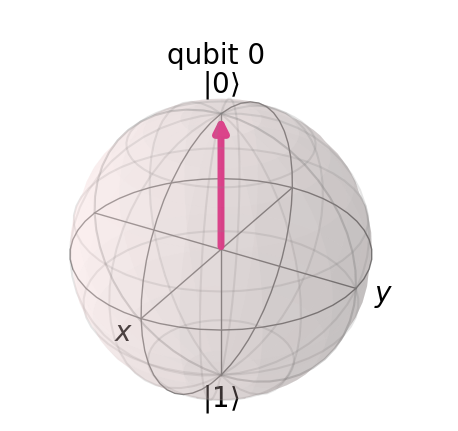#### 快速练习

1.将H门转换为$|0⟩$、$|1⟩$、$|+⟩$和$|-⟩$外积的形式。

2.表明将门序列:HZH应用于任何量子比特状态等同于应用X门。

3.找到与Y门等价的X, Z和H门的组合(忽略全局相位)。

## 4.题外话：以不同的基测量

$$p(|+\rangle) = |\langle+|q\rangle|^2, \quad p(|-\rangle) = |\langle-|q\rangle|^2$$

# Create the X-measurement function:
def x_measurement(qc, qubit, cbit):
"""Measure 'qubit' in the X-basis, and store the result in 'cbit'"""
qc.h(qubit)
qc.measure(qubit, cbit)
return qc

initial_state = [1/sqrt(2), -1/sqrt(2)]
# Initialize our qubit and measure it
qc = QuantumCircuit(1,1)
qc.initialize(initial_state, 0)
x_measurement(qc, 0, 0)  # measure qubit 0 to classical bit 0
qc.draw()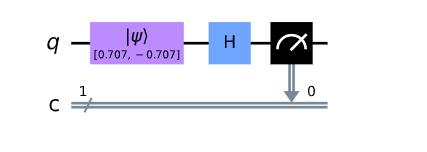$$X = HZH$$

# $$HZH = \tfrac{1}{\sqrt{2}}\begin{bmatrix} 1 & 1 \ 1 & -1 \end{bmatrix} \begin{bmatrix} 1 & 0 \ 0 & -1 \end{bmatrix} \tfrac{1}{\sqrt{2}}\begin{bmatrix} 1 & 1 \ 1 & -1 \end{bmatrix} \begin{bmatrix} 0 & 1 \ 1 & 0 \end{bmatrix} =X$$

qobj = assemble(qc)  # Assemble circuit into a Qobj that can be run
counts = sim.run(qobj).result().get_counts()  # Do the simulation, returning the state vector
plot_histogram(counts)  # Display the output on measurement of state vector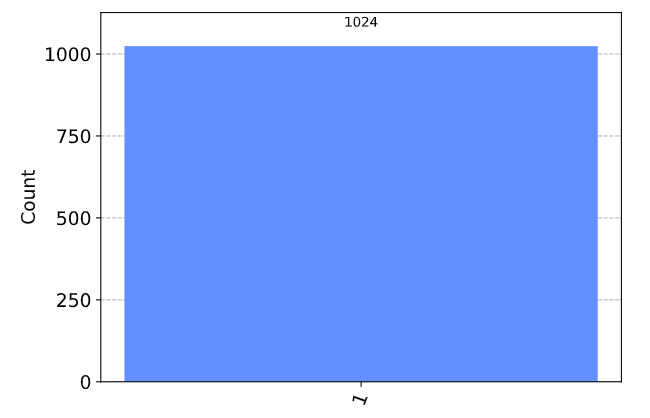#### 快速练习

1.如果我们在状态中初始化我们的量子比特$|+⟩$，在状态下测量它的概率是多少$|-⟩$?

2.使用Qiskit测量一个$|0⟩$量子比特显示在状态$|+⟩$和$|-⟩$的概率(提示:您可能想使用。get_counts()plot_histogram())。

3.试着创建一个以Y为基的函数。

H门的引入使我们能够探索一些有趣的现象，但我们在量子操作方面仍然非常有限。现在让我们介绍一种新型的门:

## 5. P门

P门(相位门)是参数化的，也就是说，它需要一个数字(ϕ)来告诉它具体做什么。P-门围绕Z轴方向旋转ϕ。它的形式为矩阵:
$$P(\phi) = \begin{bmatrix} 1 & 0 \ 0 & e^{i\phi} \end{bmatrix}$$
ϕ是实数。

# Run the code in this cell to see the widget
from qiskit_textbook.widgets import gate_demo
gate_demo(gates='pauli+h+p')


qc = QuantumCircuit(1)
qc.p(pi/4, 0)
qc.draw()。实际上，本章还会提到三个更常用的P门，它们都是P门的特殊情况。

## 6. I、S和T门

### 6.1 I门

$$I = \begin{bmatrix} 1 & 0 \ 0 & 1\end{bmatrix}$$

$$I = XX$$

1.I门的本征态是什么？

### 6.2 S门

$$S = \begin{bmatrix} 1 & 0 \ 0 & e^{\frac{i\pi}{2}} \end{bmatrix}, \quad S^\dagger = \begin{bmatrix} 1 & 0 \ 0 & e^{-\frac{i\pi}{2}} \end{bmatrix}$$

$$SS|q\rangle = Z|q\rangle$$

qc = QuantumCircuit(1)
qc.s(0)   # Apply S-gate to qubit 0
qc.sdg(0) # Apply Sdg-gate to qubit 0
qc.draw()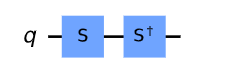### 6.3 T门

T型门是一种非常常用的门，它是带有P形门 $φ=π/4$:
$$T = \begin{bmatrix} 1 & 0 \ 0 & e^{\frac{i\pi}{4}} \end{bmatrix}, \quad T^\dagger = \begin{bmatrix} 1 & 0 \ 0 & e^{-\frac{i\pi}{4}} \end{bmatrix}$$

qc = QuantumCircuit(1)
qc.t(0)   # Apply T-gate to qubit 0
qc.tdg(0) # Apply Tdg-gate to qubit 0
qc.draw()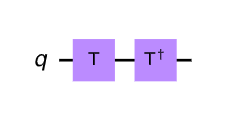# Run the code in this cell to see the widget
from qiskit_textbook.widgets import gate_demo
gate_demo()


## 7. U型门

$$U(\theta, \phi, \lambda) = \begin{bmatrix} \cos(\frac{\theta}{2}) & -e^{i\lambda}\sin(\frac{\theta}{2}) \ e^{i\phi}\sin(\frac{\theta}{2}) & e^{i(\phi+\lambda)}\cos(\frac{\theta}{2}) \end{bmatrix}$$

\begin{aligned} U(\tfrac{\pi}{2}, 0, \pi) = \tfrac{1}{\sqrt{2}}\begin{bmatrix} 1 & 1 \ 1 & -1 \end{bmatrix} = H & \quad & U(0, 0, \lambda) = \begin{bmatrix} 1 & 0 \ 0 & e^{i\lambda}\ \end{bmatrix} = P \end{aligned}

# Let's have U-gate transform a |0> to |+> state
qc = QuantumCircuit(1)
qc.u(pi/2, 0, pi, 0)
qc.draw()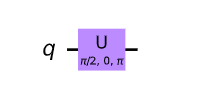# Let's see the result
qc.save_statevector()
qobj = assemble(qc)
state = sim.run(qobj).result().get_statevector()
plot_bloch_multivector(state)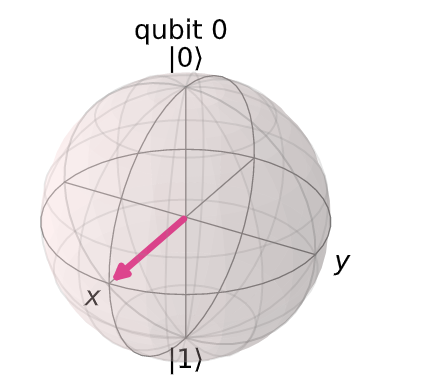## 8. 额外的资源

import qiskit.tools.jupyter
%qiskit_version_table


#### 版本信息

Qiskit Software Version
qiskit-terra 0.22.3
qiskit-aer 0.11.1
qiskit-ibmq-provider 0.19.2
qiskit 0.39.3
System information
System information 3.7.12
Python compiler GCC 9.4.0
Python build default, Oct 26 2021 06:08:53
OS Linux
CPUs 4
Memory (Gb) 51.00146484375